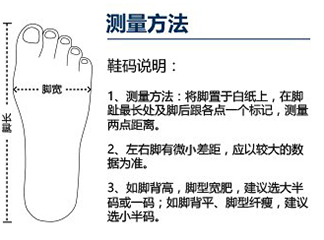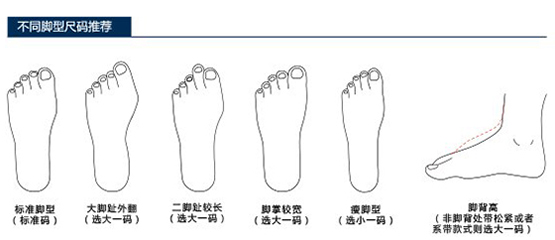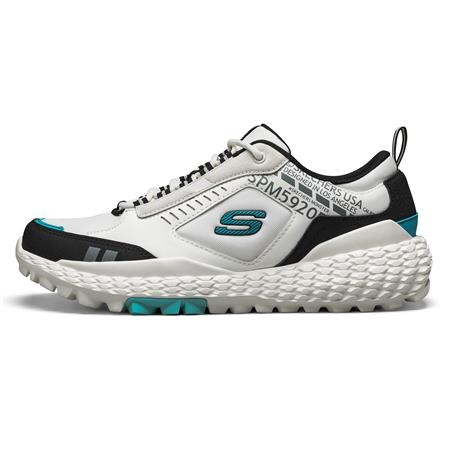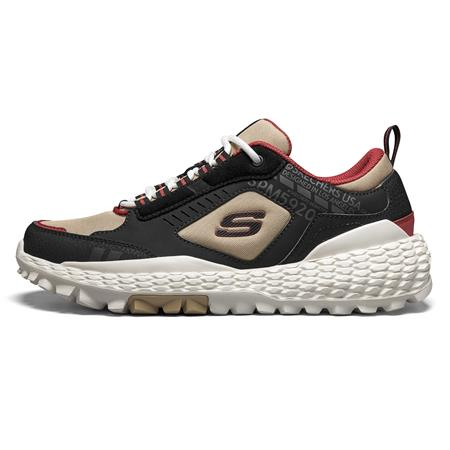# MONSTER

HK\$999.00

## Product ActionsHK\$999.00

## Promotions

### 尺碼對照表

6.5
7
7.5
8
8.5
9
9.5
10
10.5
11
11.5
12

39=39
39.5=39.5
40=40
41=41
41.5=42
42=42.5
42.5=43
43=43.5
44=44
45=44.5
45.5=45
46=45.5

24.5
25
25.5
26
26.5
27
27.5
28
28.5
29
29.5
30
••### 產品詳細信息### 尺碼對照表

6.5
7
7.5
8
8.5
9
9.5
10
10.5
11
11.5
12

39=39
39.5=39.5
40=40
41=41
41.5=42
42=42.5
42.5=43
43=43.5
44=44
45=44.5
45.5=45
46=45.5

24.5
25
25.5
26
26.5
27
27.5
28
28.5
29
29.5
30
••### 產品詳細信息